# Quiz 8: Labor Mobility

Business

If X and Y are two places where a person is living and would like to live in future respectively, then the present value (PV) of income at places X and Y with the wage rate (w and w') and discount rate(r) can be calculated as below :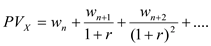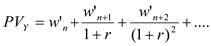M=Migration cost Therefore the net gain to migration would be :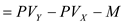If this equation gives a positive result, then only the migration would be beneficial otherwise the labor should continue with the present job and location. The highest migration cost that the worker would be willing to take would be the point where the net present value of wages is equal to migration cost (net gain is equal to zero). If the net present value is greater than migration cost then the worker would not migrate. If a worker gets \$20,000 in Pennsylvania and \$22,000 if she/he moves to Illinois, then at a discount rate of 10%, the maximum migration cost that the worker could incur is equal to: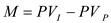.Where I and P represents Illinois and Pennsylvania.The maximum migration cost that the worker is willing to take would be \$5472.

The international labor mobility depends mainly on three factors: - Net present value of continuing in the job at same place - Net present value of switching the job and location - The marginal cost of migration Labors evaluate their net present value before taking the decision of changing location for better opportunities. This can be expressed in the form of equation. If X and Y are two places where a person is living and would like to live in future respectively, then the present value (PV) of income at places X and Y with the wage rate (w and w') and discount rate(r) can be calculated as below :M=Marginal cost Therefore the net gain to migration would be: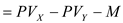If this equation gives a positive result, then only the migration would be beneficial otherwise the labor should continue with the present job and location.

If high wage worker are more likely to move to a new state for a better job, then the following points hold true: (A)The migration of high wage worker is solely due to wages. In other words, the new state provides better wages to skilled labors than the origin state. The movement of skilled labor would be towards a high paying job. (B)The migration of a worker from one place to other depends on three factors: - Net present value of continuing the present job - Net present value of switching the job and location - The marginal cost of migration Labors evaluate their net present value before taking the decision of changing location for better opportunities. The labors will migrate if the net present value of wages is greater than the migration cost. If X and Y are two places where a person is living and would like to live in future respectively, then the present value (PV) of income at places X and Y with the wage rate (w and w') and discount rate(r) can be calculated as below :M=Marginal cost Therefore the net gain to migration would be:If this equation gives a positive result, then only the migration would be beneficial otherwise the labor should continue with the present job and location. If the worker moves even when the migration cost is high, this means the new wage that is offered in new state would be much higher.